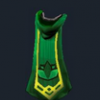# Some very interesting figures on magic defence bonus, spectral sucks.

## Recommended Posts

Three dice are rolled every attack: the attack die' date=' the strength die, and the opponent's defense die. If the attack die is greater than the defense die, the hit lands and the strength die is rolled. The strength die determines how much is damage is done. The max rolls of each die can be determined using the following formulae:

Max attack roll (MAR):

[img']http://i43.tinypic.com/2dh9wk1.png[/img]Max defense roll (MDR):These are then multiplied by 10 and floored.

The style variable can be filled with 3 for accurate or defensive styles, or 1 for controlled styles.

The void variable can be filled with 1.1 for melee and range and 1.3 for magic.

The prayer variable can be filled with the bonus listed in-game.

The turmoil variable can be filled with the total bonus (as listed in-game) minus 1.15.

Example: If the bonus is listed as 1.35 in-game, the prayer variable would be equal to 1.15 and the turmoil variable would be 20.

Paleas also found that max attack roll can be multiplied by the following, when applicable:

Melee:

Abyssal whip: multiply by 1.1

Abyssal vine whip: multiply by 1.25

Barrelchest anchor: multiply by 2

Dragon dagger: multiply by 1.1

Dragon longsword: multiply by 1.1

Dragon slayer gloves: multiply by 1.1

(Full) slayer helmet, Black mask: multiply by 7/6

Salve amulet: multiply by 7/6

Salve amulet (e): multiply by 1.2

Ranged:

Full slayer helmet, Focus sight: multiply by 7/6

Magic longbow: always 100% accuracy

Magic shortbow: multiply by 1.1

Sharpshooter aura: multiply by 1.03

Greater sharpshooter aura: multiply by 1.05

Magic:

Full slayer helmet, Hexcrest: multiply by 7/6

Runic accuracy aura: multiply by 1.03

Greater runic accuracy aura: multiply by 1.05

Thus, we can assume the following, where A is the max attack roll, and D is the max defense roll:If the attack hits, the damage dealt must be determined. This is equal to the strength roll. The max strength roll can be determined with the following formulae:

Max Strength Roll (MSR):The style variable can be filled with 3 for aggressive styles, or 1 for controlled styles.

The void variable can be filled with 1.1 for melee and range. Round down one extra time if using void range.

The prayer variable can be filled with the bonus listed in-game.

The turmoil variable can be filled with 9 if turmoil is active.

Next, multiply by bonus damage, as determined by Obliv:

~MELEE SPECIAL ATTACKS AND BONUSES~

Armadyl Godsword: Multiply by 1.375 (1.25*1.10).

†Balmung: Multiply by 1.45.

Bandos Godsword: Multiply by 1.21 (1.10*1.10).

Berserker Necklace + Obsidian Weapon: Multiply by 1.20.

Castle Wars Bracelet: Multiply by 1.20.

Dharok Set Effect: Increase the damage by an equal proportion to the proportion of Life Points lost. For example, if you have 99/990 LP (90% life lost), multiply by 1.90.

Dragon Claws: Set M equal to your max hit. Where [[x]] means to round x down, your hits are: [[M]] + [[M/2]] + [[M/4]] + [[M/4 + 1]]. The maximum damage will be 2M±1.

Dragon Dagger: Multiply by 1.15.

†Dragon Halberd: Multiply by 1.10.

†Dragon Longsword: Multiply by 1.25.

†Dragon Mace: Multiply by 1.45.

*Full Slayer Helmet: Multiply by 7/6.

Keris: Multiply by 3. †When you do not puncture a hole in your opponent's chitin with the special, the damage increase is still approximately 25% (ONLY versus appropriate enemies).

Korasi's Sword: Multiply by 1.50.

†Rune Claws: Multiply by 1.10.

*Salve Amulet: Multiply by 7/6.

*Salve Amulet(e): Multiply by 1.20.

*Slayer Helmet: Multiply by 7/6.

Statius's Warhammer: Multiply by 1.25.

Vesta's Longsword: Multiply by 1.20.

Zamorak Godsword: Multiply by 1.10.

~RANGED SPECIAL ATTACKS AND BONUSES~

Dark Bow, with Dragon Arrows: Multiply by 1.50.

Dark Bow, without Dragon Arrows: Multiply by 1.30.

†Diamond Bolts: Multiply by 1.15.

†Dragon Bolts: Multiply by 1.45.

Focus Sight: Multiply by 7/6.

Full Slayer Helmet: Multiply by 7/6.

Guthix Bow: Multiply by 1.50.

Kayle's Sling: Multiply by 0.90.

Morrigan's Throwing Axe: Multiply by 1.20.

†Onyx Bolts: Multiply by 1.20.

†Opal Bolts: Multiply by 1.25.

Sling: Multiply by 0.90.

Zanik's Crossbow: Add 150 to the damage, +10 more per god item your opponent wields.

Zamorak Bow: Multiply by 2.00.

~OTHER SPECIAL ATTACKS AND BONUSES~

Fire Cape: Add 40. (Ice Strykewyrms only)

* The black mask and salve amulet do not stack. Use only the black mask if both are available.

† Denotes modifiers that are neither rigorously tested nor stated in the manual.

All of this information is nice, but we need a way put all of it together. The best way is using a unit called damage per second (DPS), which can be derived using the following formula:The AD (Average Damage) variable can be filled with MSR/2 or (MSR+1)/2. There is no way for us to know whether or not it is possible to roll a 0 on the strength die, due to the inability to achieve a -64 strength bonus.

The CD (Cool Down) variable can be filled with the time (in seconds) between attacks.

From my post here:

http://www.xp-waste.com/post15978.html#p15978

##### Share on other sites

Thanks, I'll read all that over soon. :)

Cabbage! (:<

## Create an account

Register a new account